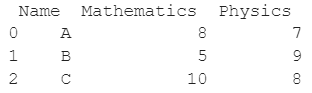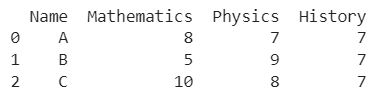# How To Add A Column To A Pandas DataFrame With A Constant Value?

A DataFrame is the primary data structure of the Pandas library and is commonly used for storing and working with tabular data. A common operation that could be performed on such data is getting the row count of an existing DataFrame in order to gauge exactly how many data points we have.

To start working with Pandas, we first need to import it into Python code:

### Python 3 Code :

``import pandas as pd``

## Running Example

Let us understand this operation with the help of an example. Consider the following DataFrame containing 3 students with names A, B, and C and their corresponding marks (out of 10) for two subjects, Mathematics and Physics.Code snippet for generating the above DataFrame :

### Python 3 Code :

``````import pandas as pd

# Dictionary for our data
data = {'Name' : ['A', 'B', 'C'], 'Mathematics' : [8, 5, 10], 'Physics' : [7, 9, 8]}

# DataFrame for the dictionary
df = pd.DataFrame(data)

# Printing the DataFrame
print(df)``````

Here, data is a dictionary we created to initialize the DataFrame. For this, we use the DataFrame() function of the Pandas library which takes the dictionary as an argument and returns the required DataFrame.

Now let us say we need to add another column to this DataFrame with the column label History and that this new column should contain a constant value, say 7, for all rows.

Therefore, the expected output i.e. the updated DataFrame should be :Let us look at different ways of performing this operation :

## Add A Column To A Pandas DataFrame With A Constant Value Using a list

In this method, we add a column to a Pandas DataFrame with a constant value by assigning a list to this new column. The list is manually generated, [7,7,7] here. We create a new column and specify the desired label, History here. df[‘History’] is used to refer to this new column.

### Python 3 Code :

``````import pandas as pd

# Dictionary for our data
data = {'Name' : ['A', 'B', 'C'], 'Mathematics' : [8, 5, 10], 'Physics' : [7, 9, 8]}

# DataFrame for the dictionary
df = pd.DataFrame(data)

# Adding a new column History with a constant value of 7 to the DataFrame df
df['History'] = [7,7,7]

# Printing the updated DataFrame
print(df)``````

Output :## Add A Column To A Pandas DataFrame With A Constant Value Using a for loop to generate list

In this method, we add a column to a Pandas DataFrame with a constant value by assigning a list to this new column. We create a new column and specify the desired label, ‘History’ here. df[‘History’] is used to refer to this new column.

The required list [7,7,7] is generated using a for loop here instead of manually listing it as in the previous method.

The _(underscore) is simply used as a throwaway variable here since there is no use for the values enumerated by the for loop

The range(len(df)) function makes the loop run from 0 to the value given by len(df) - 1. len(df) gives us the row count of the DataFrame. Therefore, our for loop here runs from 0 to 2 and returns the list as [7,7,7], as desired.

### Python 3 Code :

``````import pandas as pd

# Dictionary for our data
data = {'Name' : ['A', 'B', 'C'], 'Mathematics' : [8, 5, 10], 'Physics' : [7, 9, 8]}

# DataFrame for the dictionary
df = pd.DataFrame(data)

# Adding a new column History with a constant value of 7 to the DataFrame df
df['History'] = [7 for _ in range(len(df))]

# Printing the updated DataFrame
print(df)``````

Output :## Add A Column To A Pandas DataFrame With A Constant Value Using the DataFrame.assign() function

In this method, we add a column to a Pandas DataFrame with a constant value by using the DataFrame.assign() function. We do this by passing the parameter as the column label, ‘History’ here, and assigning the desired constant value to it, 7 here.

This function returns a copy of the DataFrame with the new column added and does not add the new column in place so we need to reassign the DataFrame returned by this function to the original DataFrame object.

### Python 3 Code :

``````import pandas as pd

# Dictionary for our data
data = {'Name' : ['A', 'B', 'C'], 'Mathematics' : [8, 5, 10], 'Physics' : [7, 9, 8]}

# DataFrame for the dictionary
df = pd.DataFrame(data)

# Adding a new column History with a constant value of 7 to the DataFrame df
df = df.assign(History = 7)

# Printing the updated DataFrame
print(df)``````

Output :## Add A Column To A Pandas DataFrame With A Constant Value Using the DataFrame.apply() function

In this method, we add a column to a Pandas DataFrame with a constant value by using the DataFrame.apply() function. We create a new column and specify the desired label, ‘History’ here. df[‘History’] is used to refer to this new column.

We use the lambda function to pass an anonymous function as a parameter to the DataFrame.apply() function. The return expression for this function is set as 7, the desired constant.

The parameter axis is assigned 1 to specify that we need to apply this function row-wise, to create a new column. This function can also be termed as a constant function.

### Python 3 Code :

``````import pandas as pd

# Dictionary for our data
data = {'Name' : ['A', 'B', 'C'], 'Mathematics' : [8, 5, 10], 'Physics' : [7, 9, 8]}

# DataFrame for the dictionary
df = pd.DataFrame(data)

# Adding a new column History with a constant value of 7 to the DataFrame df
df['History'] = df.apply(lambda x : 7, axis = 1)

# Printing the updated DataFrame
print(df)``````

Output :## Add A Column To A Pandas DataFrame With A Constant Value Using the assignment statement

In this method, we add a column to a Pandas DataFrame with a constant value by using a simple assignment. This method is most suitable for modern versions of Pandas

We create a new column and specify the desired label, ‘History’ here. df[‘History’] is used to refer to this new column. We assign the desired constant value to it, 7 here, to add a new column.

### Python 3 Code :

``````import pandas as pd

# Dictionary for our data
data = {'Name' : ['A', 'B', 'C'], 'Mathematics' : [8, 5, 10], 'Physics' : [7, 9, 8]}

# DataFrame for the dictionary
df = pd.DataFrame(data)

# Adding a new column History with a constant value of 7 to the DataFrame df
df['History'] = 7

# Printing the updated DataFrame
print(df)``````

Output :## Conclusion

In this topic, we have learned to add a column to an existing Pandas DataFrame with a constant value, following a running example of test scores of students in different subjects, thus giving us an intuition of how this concept could be applied in real-world situations. Feel free to reach out to [email protected] in case of any suggestions.

Trending